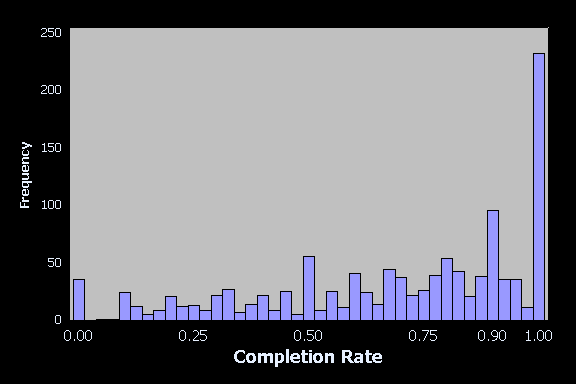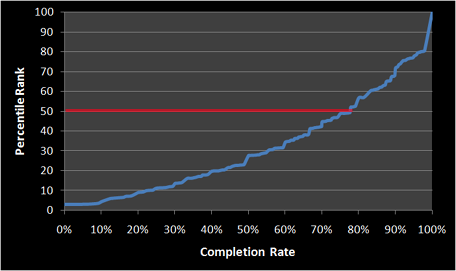It depends (you saw that coming).

Context matters in deciding what a good completion rate is for a task, however, knowing what other task completion rates are can be a good guide for setting goals.

An analysis of almost 1200 usability tasks shows that the average task-completion rate is 78%.

### The Fundamental Usability Metric

A binary task completion rate is one of the most fundamental of usability metrics. You define a scenario with clear success criteria and have the users attempt it on an application. If they complete the task successfully you give them a 1 and if they fail it you given them a 0.

After testing all your users, the average of all those 1’s and 0’s gets you the task completion rate. For example, if 9 out of 10 users complete a task, the completion rate is .90 or 90% when expressed as a percentage. What exactly is a good task completion rate?

### Context Matters

If the costs for task failure are high (loss of money, loss of life) then you need to aim for 100%. If the consequences are less impactful, then you can relax the rate a bit. I’ve often seen walk-up-and-use consumer web-applications with a 70% completion rate target. That is, a task is considered good enough for now if there is evidence from testing that at least 70% completed the task on their first attempt.

Of course, just because 70% of your sample completes the task doesn’t mean the entire user population will. You need to generate confidence intervals around your completion rate to see the likely range of the population completion rate. For example, if 10 out of 10 users complete a task, you can be 95% confident between 75% and 100% of all users will be able to complete it.

Because the lower boundary of the confidence interval exceeds 70%, you can be at least 95% confident 70% or more users will be able to complete the task. You can use this graphical calculator to see how what the minimum sample size is to have the lower end of the confidence interval exceed your goal.

### But what do you set as your goal?

Even though context matters, it can help to have an idea about how well your task completion rate stacks up against others taken during a usability test. I’ve been collecting task-completion rate data for several years—from business software, consumer software and websites.

In total I have data from 1189 tasks taken from both lab-based (90%) and unmoderated usability tests (10%). The data comes from 115 usability tests from 3472 users across over a dozen organizations. I used this data extensively in the popular report “How to Conduct a Quantitative Usability Test.

I’ve graphed the distribution of task-completion rates in a histogram below.Figure 1: Distribution of 1189 tasks at various completion rates.

When you look at the distribution of task-completion rates in the figure above, you can see there is a spread with a few noticeable spikes at 100%, 90%, 50% and 0%. We can also see that there is a positive skew with most of the completion rates coming above 50%. So right away this data shows us that 50% is not an average completion rate.

By counting the number of tasks at each completion rate from 0 to 100, I generated a cumulative distribution to more easily describe the frequency of tasks. For example, 232 tasks (20%) had completion rates of 100% and 564 (44%) had completion rates between 80 and 100%. The graph of the distribution is shown below.Figure 2: Cumulative distribution of 1189 tasks at various completion rates. An average completion rate
(50th percentile) is a 78%–shown as the red line in the figure.

### 78% is an average completion rate

With this distribution we can see that half the tasks had completion rates above 78%. So this is one threshold of good and bad–with anything above a 78% being above average. If you’re scratching your head looking for a benchmark for a task, using 78% would be a good place to start. To have a completion rate in the top quartile (75%) you’d want a completion rate above 92%. A completion rate less than 49% puts you in the bottom quartile (25%).

There are likely a few reasons for the inflated completion rates. First, many of the usability tests in the database are conducted on commercially available software–so we expect many interaction problems to be reduced. Second, users are likely trying harder than they would to complete the tasks because they are both being observed and assume there’s a point to all that toil. This is something Rich Cordes at IBM called the “if you’re asking me to do it must be possible” bias.

### Estimate the percentile of a task-completion rate

I was able to model the exponential nature of the cumulative distribution using a quadratic regression equation which fits well to the observed data (adj-R2 = 99.5%). If you have a completion rate and want to know how well it stacks up, you can interpolate using the graph above or enter your completion rate in the form below and get an approximate percentile rank. For example, a task completion rate of an 80% is better than 56% of all tasks.

A task completion rate of % is better than

56%